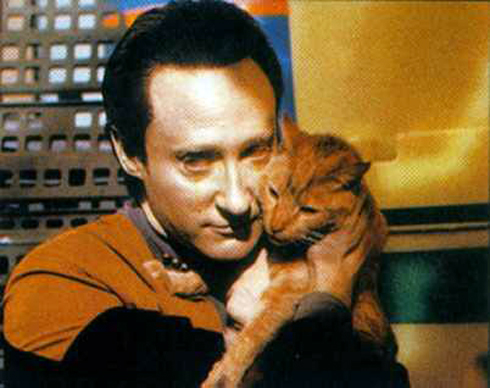by

# Making HTML Color ASCII Art with MATLAB

On one warm spring afternoon during grad school when I should have been outside enjoying nature, I stayed in the lab and wrote the following MATLAB script. It will take any 8-bit RGB image and convert it to colored HTML text; any text. As an example of its application I have emblazoned my favorite positronic life form with his ode to his cat.

``````% MATLAB script for converting an image into html color ascii art
clear;
close all;
home;
iname = 'dataandspot.jpg'; % name of original image
R = I(:,:,1); G = I(:,:,2); B = I(:,:,3);
I = uint8(I); A = 'string';
word = 'FELIS CATUS IS YOUR TAXONOMIC NOMENCLATURE, AN ENDOTHERMIC QUADRUPED CARNIVOUROUS BY NATURE; YOUR VISUAL, OLFACTORY, AND AUDITORY SENSES, CONTRIBUTE TO YOUR HUNTING SKILLS AND NATURAL DEFENSES. I FIND MYSELF INTRIGUED BY YOUR SUBVOCAL OSCILLATIONS, A SINGULAR DEVELOPMENT OF CAT COMMUNICATIONS, THAT OBVIATES YOUR BASIC HEDONISTIC PREDILECTION, FOR A RHYTHMIC STROKING OF YOUR FUR TO DEMONSTRATE AFFECTION. A TAIL IS QUITE ESSENTIAL FOR YOUR ACROBATIC TALENTS; YOU WOULD NOT BE SO AGILE IF YOU LACKED ITS COUNTERBALANCE. AND WHEN NOT BEING UTILIZED TO AIDE IN LOCAMOTION, IT OFTEN SERVES TO ILLUSTRATE THE STATE OF YOUR EMOTION. O SPOT, THE COMPLEX LEVELS OF BEHAVIOUR YOU DISPLAY, CONNOTE A FAIRLY WELL-DEVELOPED COGNITIVE ARRAY. AND THOUGH YOU ARE NOT SENTIENT, SPOT, AND DO NOT COMPREHEND, I NONETHELESS CONSIDER YOU A TRUE AND VALUED FRIEND. ';
wlen = length(word);
% colors are read in as RGB and must be converted to hexadecimal for use in
% html R G B
% hex characters are 0 1 2 3 4 5 6 7 8 9 A B C D E F (base 16) - 0-255 to
% 00-FF maxascii = 200;
% maximum number of ascii characters to use in width direction
[r,c,dummy] = size(I);
% image is black and white so these numbers will be between 1 and 255
maxdim = max(r,c); % size of ascii output
heightcompfactor = 1.25;
% height contraction
heightcompfactor = 1.1;
% font size 10
if c > maxascii
factor = c./maxascii;
else factor = 1.12;
end
nr = floor(r/(heightcompfactor*factor));
nrstar = floor(nr/wlen)*wlen+1;
nc = floor(c/factor);
hexColor = {};
for i = 1:nc
for j = 1:nr
Rmean = round(mean(mean(R(round((j-1)*factor*heightcompfactor+1):round(j*factor*heightcompfactor),round((i-1)*factor+1):round(i*factor)))));
Gmean = round(mean(mean(G(round((j-1)*factor*heightcompfactor+1):round(j*factor*heightcompfactor),round((i-1)*factor+1):round(i*factor)))));
Bmean = round(mean(mean(B(round((j-1)*factor*heightcompfactor+1):round(j*factor*heightcompfactor),round((i-1)*factor+1):round(i*factor)))));
hexColor{j,i,1}=dec2hex(Rmean,2);
hexColor{j,i,2}=dec2hex(Gmean,2);
hexColor{j,i,3}=dec2hex(Bmean,2);
end
end
% write HTML encoding of image to a file
fid = fopen('asciicolor.html','a');
fprintf(fid,'%s\n','<html>');
fprintf(fid,'%s\n','pre{font-size:8pt;font-family:monospace;line-height:7pt}');
fprintf(fid,'%s\n','<pre><b>');
kk = 0;
for j = 1:size(hexColor,1); % for all rows
for i=1:size(hexColor,2); % for all columns
kk = kk+1;
curletter = word(mod(kk-1,wlen)+1);
fprintf(fid,'%s',['<font color=#' hexColor{j,i,1} hexColor{j,i,2} hexColor{j,i,3} '>' curletter '</font>']);
end
fprintf(fid,'%s\n','');
end
fprintf(fid,'%s\n','</span></b></pre></body></html>');``````

### Original ImageOde To Spot

### 1 Comment

1.